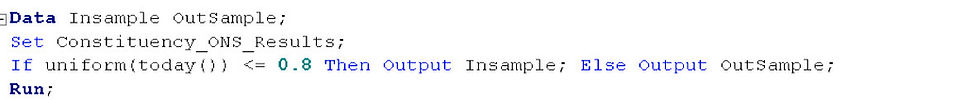top of page
Search

# Decision Trees in Base SAS

As it is topical I thought I would look at election results. I have taken a dataset of demographic information by parliamentary constituency from the ONS. To this I have attached the party that won this constituency in the 2010 general election. Using a decision tree I am going to see if it is possible to predict the outcome of the election using just the ONS data.

SAS comes with a very useful procedure called Hpsplit which can be used to create a decision tree. I am going to use the entropy method which is a common way of creating a decision tree. The entropy method works by splitting the data in the way that creates the most differentiation at each level. So a split that halves the data would be considered better than one that splits it into segments with a quarter and three quarters.

First I split the data into an in sample and an out sample:Next I run the Hpsplit procedure on the in sample. There are a few setting here which need explanation. The maxbranch setting decides how many branches each line of the tree can split into. Here I have set this to 2 so each line in the tree is a Boolean yes / no decision. The maxdepth setting says how many rows the tree can have. I have set this to 5 which is adequate for this sort of problem but it could be much more. On the input line I have set the level as equal to int. This tells SAS that all of the factors are continuous and not discrete.The procedure has produced a dataset called TreeInformation. This has one line for each of the decisions in the tree. There is a field called ID which uniquely numbers each decision and a field called parent which refers to the decision before this one. Therefore creating a logical chain of decisions through the tree. The factor that the data is split on the each decision is in a field called insplitvar and the decision is called simply decision. I want to apply this tree back on the out of sample to see how predictive it is. This requires a bit of reformatting of the dataset because I want to end up with a dataset that has each unique journey thorough the tree as a line and stores the SAS code for making the journey plus the prediction for this journey.

So I create a dataset for each level in the tree.Next I combine these datasets so that I get one line for each journey with each decision in this journey numbered.There is not always a decision at each depth because some journeys end before the fifth line so I just set these to holding values of 1 = 1.So I read the contents of this dataset into macro variables. These will be used in If statements to determine each prediction.Next I apply these logic statements to the out sample. This creates a prediction for each constituency in the out sample.Finally I test to see how many constituencies in the out sample are correctly predicted.This particular tree has predicted about 75% of the out sample constituencies correctly. Pretty good. Next week I will expand on these macros to create a random forest which will further boost the predictiveness.